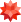# Online Integral Calculator

## More than just an online integral solver

Wolfram|Alpha is a great tool for calculating antiderivatives and definite integrals, double and triple integrals, and improper integrals. It also shows plots, alternate forms, and other relevant information to enhance your mathematical intuition.## Tips for entering queries

Enter your queries using plain English. To avoid ambiguous queries, make sure to use parentheses where necessary. Here are some examples illustrating how to ask for an integral.

## What are integrals?

Integration is an important tool in calculus that can give an antiderivative or represent area under a curve.

The indefinite integral of f(x), denoted int f(x)\ dx, is defined to be the antiderivative of f(x). In other words, the derivative of int f(x)\ dx is f(x). Since the derivative of a constant is zero, indefinite integrals are defined only up to an arbitrary constant. For example, int sin(x)\ dx = -cos(x) + "constant", since the derivative of -cos(x) + "constant" is sin(x). The definite integral of f(x) from x = a to x = b, denoted int_(a)^(b) f(x)\ dx, is defined to be the signed area between f(x) and the x axis, from x = a and x = b.

Both types of integrals are tied together by the fundamental theorem of calculus. This states that if f(x) is continuous on [a,b] and F(x) is its continuous indefinite integral, then int_(a)^(b) f(x)\ dx = F(b) - F(a). This means int_(0)^(pi) sin(x)\ dx = (-cos(pi))-(-cos(0)) = 2. Sometimes an approximation to a definite integral is desired. A common way to do so is to place thin rectangles under the curve and add the signed areas together. Wolfram|Alpha can solve a broad range of integrals.

## How Wolfram|Alpha calculates integrals

Wolfram|Alpha computes integrals differently than people. It calls Mathematica's Integrate function, which represents a huge amount of mathematical and computational research. Integrate doesn't do integrals the way people do. Instead, it uses powerful, general algorithms that often involve very sophisticated math. There are a couple of approaches that it most commonly takes. One involves working out the general form for an integral, then differentiating this form and solving equations to match undetermined symbolic parameters. Even for quite simple integrands, the equations generated in this way can be highly complex and require Mathematica's strong algebraic computation capabilities to solve. Another approach that Mathematica uses in working out integrals is to convert them to generalized hypergeometric functions, then use collections of relations about these highly general mathematical functions.

While these powerful algorithms give Wolfram|Alpha the ability to compute integrals very quickly and handle a wide array of special functions, understanding how a human would integrate is important too. As a result, Wolfram|Alpha also has algorithms to perform integrations step by step. These use completely different integration techniques that mimic the way humans would approach an integral. This includes integration by substitution, integration by parts, trigonometric substitution, and integration by partial fractions.OTHER CALCULATORS